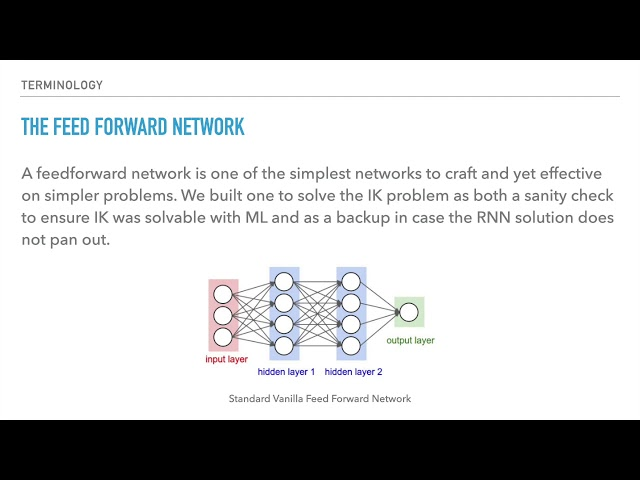# What is Kinematic modeling

##### Kinematic Modeling: Techniques and Applications in Robotics

Kinematic modeling is a technique used in robotics to describe the motion of a robot, without taking into account its dynamics. This approach is useful for a variety of applications, including robot design, control, and simulation. In this article, we'll explore the basics of kinematic modeling and its various techniques and applications.

##### The Basics of Kinematic Modeling

Kinematic modeling is based on the idea that the motion of a robot can be described by a series of interconnected links, each of which has a specific joint and orientation angle. This model is known as the robot's kinematic tree. The kinematic tree is typically represented as a set of transformation matrices, which describe the position and orientation of each link relative to its parent link.

There are several types of joints used in robotics, including:

• Revolute joints: these are joints that rotate around an axis.
• Prismatic joints: these are joints that translate along an axis.
• Spherical joints: these are joints that rotate in all directions.

The orientation of each link is described using a coordinate system, which is typically defined based on the orientation of its parent link.

##### Forward Kinematics

One of the main applications of kinematic modeling is for forward kinematics, which is the process of calculating the position and orientation of the robot's end effector (i.e. the tool or gripper at the end of the robot's arm) given the joint angles of each link in the kinematic tree.

Forward kinematics can be performed using a technique known as the DH convention (named after Denavit and Hartenberg), which involves a series of coordinate transforms to describe the position and orientation of each link relative to the base of the robot.

Another technique for forward kinematics is known as product of exponentials (POE), which involves expressing the transformation matrix for each link as a product of exponential matrices. POE is often used for robots with non-standard joint types.

##### Inverse Kinematics

Another important application of kinematic modeling is for inverse kinematics, which is the process of calculating the joint angles required to achieve a desired position and orientation of the end effector.

Inverse kinematics can be a challenging problem, as there are often multiple solutions (or no solutions) for a given end effector position and orientation. Several techniques have been developed for solving inverse kinematics, including:

• Geometric methods: these involve finding a closed-form solution for the joint angles based on the geometry of the robot.
• Numerical methods: these involve using numerical optimization techniques to find a solution that meets a set of constraints, such as joint limits and obstacle avoidance.
• Hybrid methods: these involve a combination of geometric and numerical methods to improve the performance and accuracy of the solution.
##### Kinematic Simulation

Kinematic modeling is also useful for simulating the motion of a robot before it is actually built. This allows designers and engineers to test different configurations and control strategies before investing in the physical hardware.

Kinematic simulation involves creating a digital model of the robot's kinematic tree, along with a model of the environment in which it will operate. The simulation can then be run to generate a visual representation of the robot's motion, as well as data on its performance and behavior.

Kinematic simulation is particularly useful for testing complex motions and tasks, as well as for evaluating the safety and efficiency of the robot's design.

##### Conclusion

Kinematic modeling is an important technique in robotics, providing a framework for describing the motion of a robot without considering its dynamics. This approach is used for a variety of applications, including forward and inverse kinematics, simulation, and control. By understanding the basics of kinematic modeling and its various techniques and applications, designers and engineers can create more effective and efficient robots, capable of performing complex tasks in a wide range of environments.